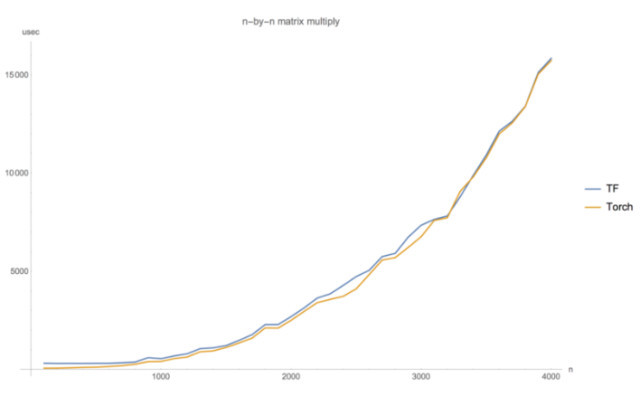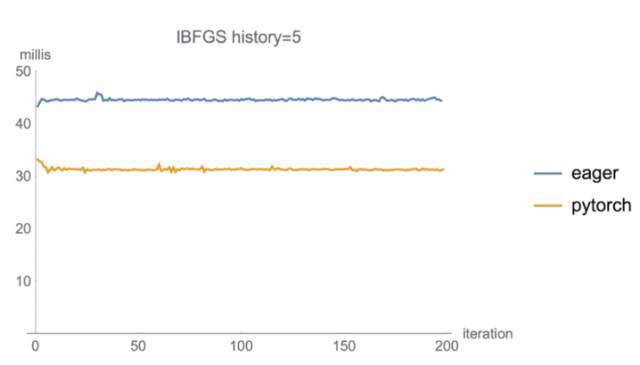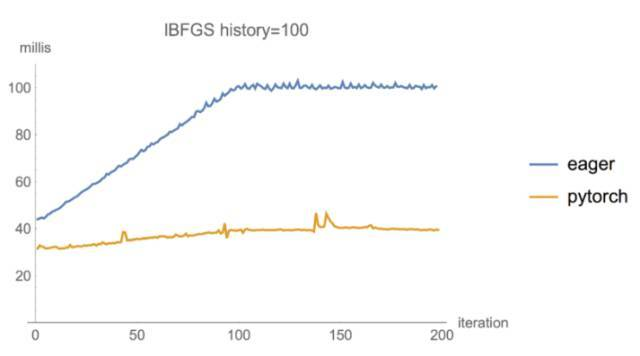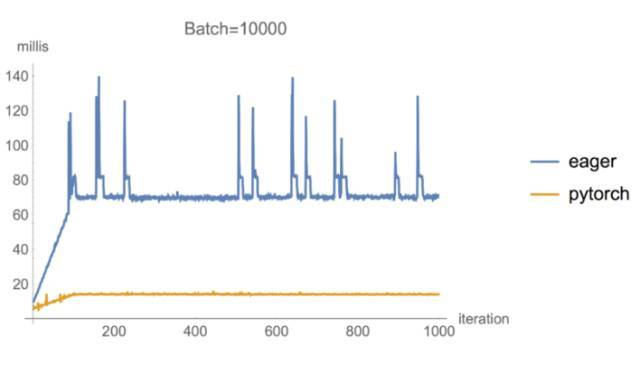Auto ByteScience AI# 终于！TensorFlow引入了动态图机制Eager Execution

PyTorch 的动态图一直是 TensorFlow 用户求之不得的功能，谷歌也一直试图在 TensorFlow 中实现类似的功能。最近，Google Brain 团队发布了 Eager Execution，一个由运行定义的新接口，让 TensorFlow 开发变得简单许多。在工具推出后，谷歌开发人员 Yaroslav Bulatov 对它的性能与 PyTorch 做了横向对比。

Eager Execution 的优点如下：

• 快速调试即刻的运行错误并通过 Python 工具进行整合
• 借助易于使用的 Python 控制流支持动态模型
• 为自定义和高阶梯度提供强大支持
• 适用于几乎所有可用的 TensorFlow 运算

Eager Execution 现在处于试用阶段，因此我们希望得到来自社区的反馈，指导我们的方向。

``````import tensorflow as tf
import tensorflow.contrib.eager as tfe
tfe.enable_eager_execution()
x = [[2.]]
m = tf.matmul(x, x)``````

``````print(m)
# The 1x1 matrix [[4.]]``````

``````a = tf.constant(12)
counter = 0
while not tf.equal(a, 1):
if tf.equal(a % 2, 0):
a = a / 2
else:
a = 3 * a + 1
print(a)``````

``````def square(x):
return tf.multiply(x, x)
print(square(3.))    # [9.]

gradients_function 的调用使用一个 Python 函数 square() 作为参数，然后返回 Python callable，用于计算输入的 square() 偏导数。因此，为了得到输入为 3.0 时的 square() 导数，激活 grad(3.0)，也就是 6。

``````gradgrad = tfe.gradients_function(lambda x: grad(x))

``````def abs(x):
return x if x > 0. else -x

``````def log1pexp(x):
return tf.log(1 + tf.exp(x))
# The gradient computation works fine at x = 0.
# [0.5]
# However it returns a `nan` at x = 100 due to numerical instability.
# [nan]``````

``````@tfe.custom_gradient
def log1pexp(x):
e = tf.exp(x)
return dy * (1 - 1 / (1 + e))
# Gradient at x = 0 works as before.
# [0.5]
# And now gradient computation at x=100 works as well.
# [1.0]``````

``````class MNISTModel(tfe.Network):
def __init__(self):
super(MNISTModel, self).__init__()
self.layer1 = self.track_layer(tf.layers.Dense(units=10))
self.layer2 = self.track_layer(tf.layers.Dense(units=10))
def call(self, input):
"""Actually runs the model."""
result = self.layer1(input)
result = self.layer2(result)
return result``````

``````# Let's make up a blank input image
model = MNISTModel()
batch = tf.zeros([1, 1, 784])
print(batch.shape)
# (1, 1, 784)
result = model(batch)
print(result)
# tf.Tensor([[[ 0.  0., ...., 0.]]], shape=(1, 1, 10), dtype=float32)``````

``````
def loss_function(model, x, y):
y_ = model(x)
return tf.nn.softmax_cross_entropy_with_logits(labels=y, logits=y_)``````

``````optimizer = tf.train.GradientDescentOptimizer(learning_rate=0.001)
for (x, y) in tfe.Iterator(dataset):

``````with tf.device("/gpu:0"):
for (x, y) in tfe.Iterator(dataset):
optimizer.minimize(lambda: loss_function(model, x, y))``````

Eager execution 使开发和调试互动性更强，但是 TensorFlow graph 在分布式训练、性能优化和生产部署中也有很多优势。

Eager execution 的使用方法对现有 TensorFlow 用户来说应是直观的。目前只有少量针对 eager 的 API；大多数现有的 API 和运算需要和启用的 eager 一起工作。请记住以下内容：

• 安装 TensorFlow 的 nightly 版本（https://github.com/tensorflow/tensorflow#installation）
• 从 eager execution 用户指南（https://github.com/tensorflow/tensorflow/tree/master/tensorflow/contrib/eager/python/g3doc/guide.md）中获取详细的指导
• 在 GitHub 中查看 eager 示例（https://github.com/tensorflow/tensorflow/tree/master/tensorflow/contrib/eager/python/examples）

Eager Execution 目前仅处于开发的前期，它的性能究竟如何？Google Brain 的工程师 Yaroslav Bulatov 对这一新工具做出了评测。TensorFlow 此前最令人诟病的问题就是它必须将计算定义为静态图。

``````pip install tf-nightly-gpu
python
from tensorflow.contrib.eager.python import tfe
tfe.enable_eager_execution()
a = tf.random_uniform((10,))
b = tf.random_uniform((10,))
for i in range(100):
a = a*a
if a>b:
break
print(i)``````

``````a = a.gpu() # copies tensor to default GPU (GPU0)
a = a.gpu(0) # copies tensor to GPU0
a = a.gpu(1) # copies tensor to GPU1
a = a.cpu() # copies tensor back to CPU``````

``````torch.sum -> tf.reduce_sum」
array.T -> tf.transpose(array) 等``````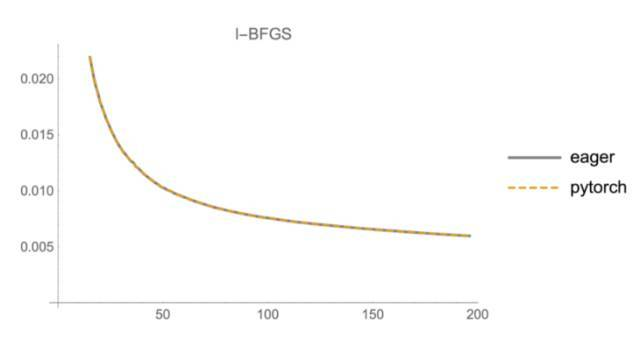``````# expensive way to compute factorial of n
def factorial(n):
def f(x):
return tf.pow(x, n)
for i in range(n):
return f(1.)``````

``````@tfe.custom_gradient
def noisy_square(x):
x = 2.
points = []
for i in range(20):
print(x, loss(x))``````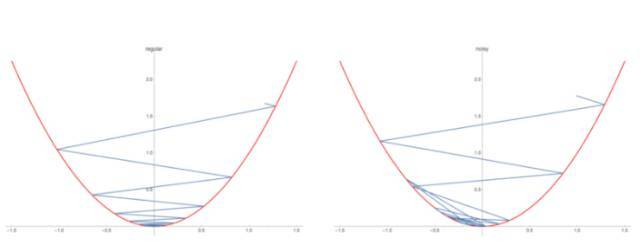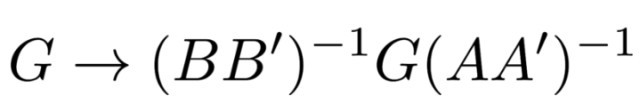``````@tfe.custom_gradient
def kfac_matmul(W, A):

Eager Execution 模式使你的程序执行慢一点或慢很多的程度取决于你的计算高运算强度的卷积还是矩阵相乘。